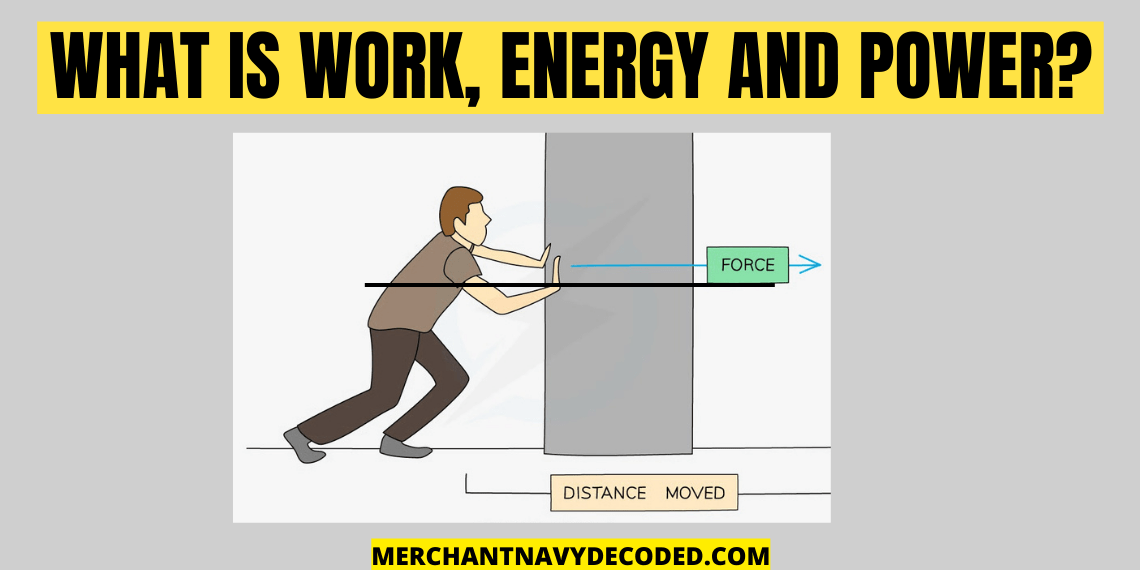## What is Work, energy and power?

Last updated on April 8th, 2023 at 09:14 am

Work: It is a measure of energy transfer that occurs when a body is displaced because of force applied on it.

If displacement is zero then work is also zero.

If the object does not move in the direction of the applied force there is no work being done even if you exert a force. Example: When you are pushing a car that is stuck in the snow.

Work=force x displacement

SI unit of work is Joules

CGS unit is Ergs

1 Joule=10^7 ergs

1 calorie= 4.184 joules

Energy: It is the capacity or ability to do work.

All forms of energy are associated with motion, for example, any given body has kinetic energy which is in motion, a tensioned device though at rest but has the potential energy for creating motion, it contains potential energy because of its configuration. For example, when a box slides down a hill the potential energy stored in it due to its elevation is converted into kinetic energy. As the box slides down the slope and comes to a halt it produces thermal heat energy. Energy can be converted from one form to another but it can neither be destroyed nor be created.

SI unit of work is Joules

CGS unit is Ergs

1 Joule=10^7 ergs

Power: Work done per unit time/rate is called power. In simple terms, it measures how quickly energy is transferred or transformed.

Power= work done / time

= Force x displacement/time

SI unit= Joules / sec = watt

CGS unit= ergs/sec

746 watt= 1 hp(Horsepower)

1000 watt= 1kw